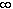#Interactive Real Analysis

Next | Previous | Glossary | Map

## 6.5. Differentiable Functions

### Examples 6.5.16(b):

FindYour browser can not handle Java applets L'Hospital's rule does not seem to apply in this case, since we have '0 *', not '0 / 0'. But if we write this expression as xn / ex we see that we can apply the second of l'Hospital's rules.
Let
f(x) = xn and g(x) = ex

Then l'Hospital's rule applies to the limit of f(x) / g(x) as x goes to infinity. In fact, taking derivatives separately, it is easy to see that we can continue to apply l'Hospital's rule n times. The n-th application of the rule will yield the expression

n! / ex
which approaches zero as x approaches infinity. Thus, applying l'Hospital's rule n times we get:= 0
Note: This is simply saying that the exponential function grows faster than any power of x as x goes to infinity. Therefore, when numerator and denominator 'race' to infinity, the denominator 'wins', forcing the fraction to be zero as x goes to infinity.
Next | Previous | Glossary | Map